# Bitwise hacks in Ruby

If you've ever thought of using Ruby to access libraries in C or Java, or to manipulate the operating system then it's critical that you know the basics of bit manipulation. We start out with the basics of binary in Ruby and finish with John Carmack's legendary inverse square root approximation.

Chances are you don't usually need to do any bitwise math in your day job. Ruby's bitwise AND and OR operators ( & and | ) are probably used more by accident than on purpose. Who hasn't accidentally typed & when they meant &&?

But if you grew up programming lower level languages like C or Assembler, or in my case Turbo Pascal then you've probably done at least some bit twiddling.

Bitwise solutions to problems are just cool. If you're able to solve a problem of yours using the most basic operations that a computer is capable of (binary math), it doesn't get any more elegant than that.

## Working with Binary in Ruby

You probably know that everything in your computer is represented as numbers, and those numbers are in binary format. But what does that look like in ruby? In this example, I'm using Ruby to look up the ASCII char code for the letter "a", then printing it as "binary."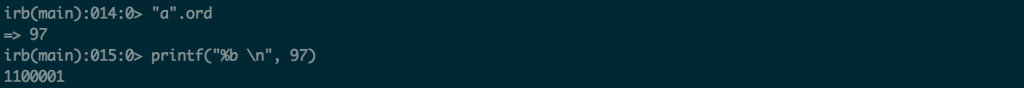You can use the `ord` method in Ruby to get a character's ASCII code, and then use `printf` to print a "binary" representation of it.

Although we have to use a method like printf to display the number in binary, it was always binary.  You can write binary numbers inside your Ruby code by using a syntax like `0b11111111`.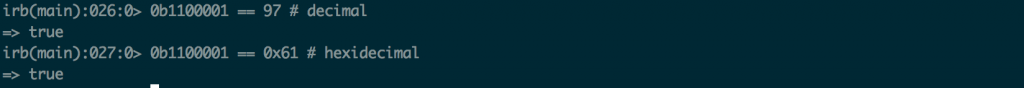To add binary literals to your code, prefix the string of ones and zeroes with 0b. So `0b11111111`

## Manipulating Binary Values

Now that we know how to use binary literals in ruby, we can start playing with them. To do that we'll use the bitwise operators.

You're probably comfortable with boolean operators like &&. The expression a && b returns true only if a and b are both true. Bitwise operators are very similar.

For example, bitwise AND takes two values and compares them bit by bit. If both bits are 1, it sets the corresponding output bit to 1. If not, it sets it to zero. So if you have eight bits, you'll have eight separate ANDs happen. If you have one bit, you have one AND. The following examples illustrate this using a single bit.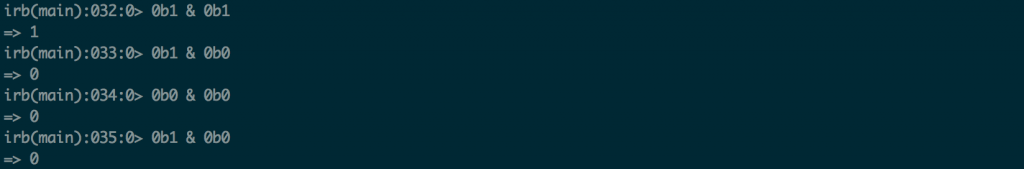Example of bitwise AND operations with a single bit.

It works the same for two bits.Each pair of bits is anded separately

## Ruby's Bitwise Operators

Pretty much every programming languages comes with this set of bitwise operators. If you're not familiar with them, spend a little time in IRB trying them out. Once you learn them, you'll be able to use them for the rest of your life.

Operator Description Example

& Bitwise AND Operator sets the result's bit to 1 if it is 1 in both input values `0b1010 & 0b0111 == 0b0010`
| Bitwise OR Operator set's the result's bit to 1 if it is 1 in either input value `0b1010 | 0b0111 == 0b1111`
^ Bitwise XOR Operator sets the result bit to 1 if it is 1 in either input value, but not both `0b1010 | 0b0111== 0b1101`
~ Bitwise Inverse operator sets result bit to 0 if the input is 1 and vise versa `~0b1010 == 0b0101`
<< Bitwise Left Shift Operator. Moves the input bits left by a specified number of places. `0b1010 << 4== 0b10100000`
>> Bitwise Right Shift Operator. Move input bits right by a certain number of places `0b1010 >> 4 == 0b0000`

## Practical Use: Configuration Flags

Ok, ok, this has to be the most boring use of bitwise math. But it's also one of the most common. If you ever have to interface with code written in Java or C or C++ you'll come across bitwise configuration flags eventually.

Imagine that it's 1996 and you just built a database system from scratch. Since you just watched the movie Hackers, you figure it might be good to build in some kind of access control.

There are 8 actions that a user can take in your DB: read, write, delete, and five more.  You want to be able to set each of them independently. You might have a user that can read but not write or delete. You might have one that can write but not drop tables.

The most efficient way to store these configuration flags is going  to be as bits in a single byte. Then a user will simply OR them together to create whatever combination of permissions they need.

``````MYDB_READ   = 0b00000001 # These numbers are called bitmasks
MYDB_WRITE  = 0b00000010
MYDB_DELETE = 0b00000100
MYDB_INDEX  = 0b00001000

``````

By the way, this is really similar to how unix file permissions are handled. If you've ever wondered why you have to use a magic number just to make  a file read-only, now you know.

It wouldn't be very useful to store configuration options in bits unless you could detect if a certain bit was set. To do that, you just use a bitwise AND.Use AND with a bitmask to determine if a bit is set.

## Less Practical Uses (Unless you're a C programmer)

Now let's do some mathe-magical stuff.

Historically, bit manipulation was used when you were trying to squeeze the last fraction of a millisecond off of some computation. So as you can imagine, it was used a lot by graphics programmers and others who needed performance above all else.

So techniques like this aren't practical for everyday Ruby development.  But they're still a fun learning exercise, and could be legitimately useful if you get into things like embedded systems programming. For a much more thorough treatment, check out this list of bit twiddling hacks.

## Multiplying & Dividing by Powers of Two

Let's look at the binary representations of the numbers 1, 2, 4, and 8. As you can see, doubling the number is equivalent to shifting all the bits one place to the left. Similarly, halving the number is the same as shifting right.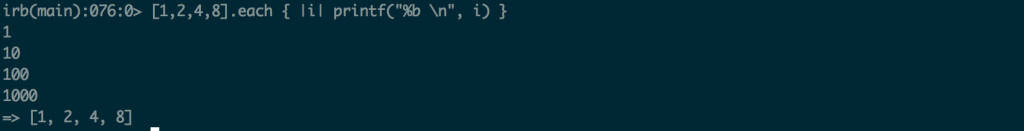Binary representations of 1, 2, 4 8

If you'll recall, we have shift-left and shift-right operators. That means we can multiply and divide by powers of two simply by shifting bits.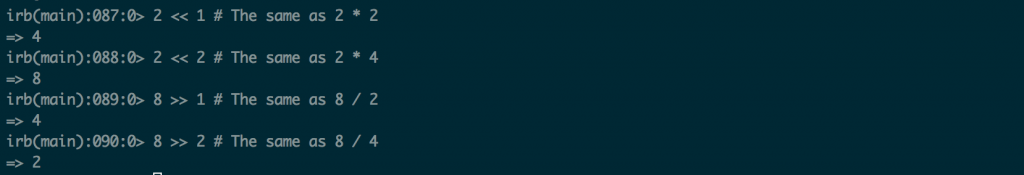You can use bitwise shift operators to multiply or divide by powers of two

## Averaging Two Positive Integers

You can do basic addition of two integers like so:  (x+y) == (x&y)+(x|y) == (x^y)+2*(x&y). To calculate the average, all you need is a little division by two, which you can get via a right shift operator.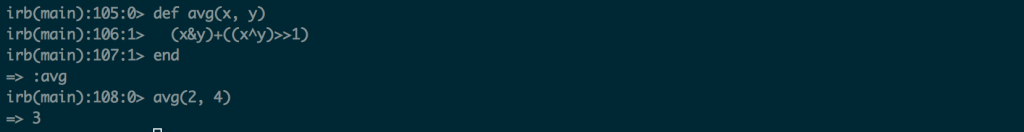You can average two positive integers like so: `(x&y)+((x^y)>>1)`

## Fast Inverse Square Root

I couldn't do a blog post on bit twiddling without including one of the most legendary examples. That is John Carmack's fast inverse square root approximation, from the 1999 Quake 3 arena source code. The algorithm isn't his, but the most famous implementation is.

I'm not going to attempt a direct port of this to Ruby, since it works by constructing a binary representation of a floating point number in a way that isn't directly translatable from C to Ruby.

``````float Q_rsqrt( float number )
{
long i;
float x2, y;
const float threehalfs = 1.5F;

x2 = number * 0.5F;
y  = number;
i  = * ( long * ) &y;                       // evil floating point bit level hacking
i  = 0x5f3759df - ( i >> 1 );               // what the fuck?
y  = * ( float * ) &i;
y  = y * ( threehalfs - ( x2 * y * y ) );   // 1st iteration
//  y  = y * ( threehalfs - ( x2 * y * y ) );   // 2nd iteration, this can be removed

return y;
}
``````

Honeybadger has your back when it counts. We're the only error tracker that combines exception monitoring, uptime monitoring, and cron monitoring into a single, simple to use platform.

Our mission: to tame production and make you a better, more productive developer. Learn more#### Starr Horne

Starr Horne is a Rubyist and Chief JavaScripter at Honeybadger.io. When she's not neck-deep in other people's bugs, she enjoys making furniture with traditional hand-tools, reading history and brewing beer in her garage in Seattle.

“We’ve looked at a lot of error management systems. Honeybadger is head and shoulders above the rest and somehow gets better with every new release.”Michael Smith
Are you using Bugsnag, Rollbar, or Airbrake for your monitoring? Honeybadger includes exception, uptime, and check-in monitoring — all for probably less than you’re paying now. Discover why so many companies are switching to Honeybadger here.
Stop digging through chat logs to find the bug-fix someone mentioned last month. Honeybadger's built-in issue tracker keeps discussion central to each error, so that if it pops up again you'll be able to pick up right where you left off.